Get ICSE Solutions for Class 10 Mathematics Chapter 8 Ratio and Proportion for ICSE Board Examinations on ICSESolutions.com. We provide step by step Solutions for ICSE Mathematics Class 10 Solutions Pdf. You can download the Class 10 Maths ICSE Textbook Solutions with Free PDF download option.

## Ratio and Proportion Class 10 Maths ICSE Solutions

### Formulae

1. When two or more ratios are multiplied together, they are said to be compounded.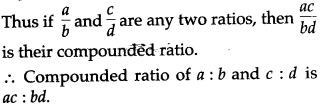2. A ratio compounded with itself is called duplicate ratio of the given ratio.3. The reciprocal ratio a : b is b : a.
4. Proportion: An equality of two ratios is called a proportion.5. The quantities a, b, c and d are called the terms of the proportion; a, b, c and d are the first, second, third and fourth terms respectively. First and fourth terms are called extremes (or extreme terms). Second and third terms are called means (or middle terms).⇒ product of extreme terms = product of middle terms.
Thus, if the four quantities are in proportion then the product of extreme terms = product of middle terms. This is called cross product rule.
6. Fourth proportional: If a, b, c and d are in proportion then d is called the fourth proportional.7. In particular, three (non-zero) quantities of the same kind, a, b and c are said to be in continued proportion iff the ratio of a to b is equal to the ratio of b to c.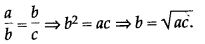8. First proportional: If a, b are c are in continued proportion, then a is called the first proportional.
Third proportional: If a, b and c are in continued proportion, then c is called the third proportional.
Mean proportional: If a, b and c are in continued proportion, then b is called the mean proportional of a and c.
Thus, if b is the mean proportional of a and c, thenHence, the mean proportion between two numbers is the positive square root of their product.

Properties of Ratio & Proportion:### Determine the Following

Question 1. Which is greater 4 : 5 or 19 : 25.Question 2. Arrange 5 : 6, 8 : 9, 13 : 18 and 7 : 12 in ascending order of magnitude.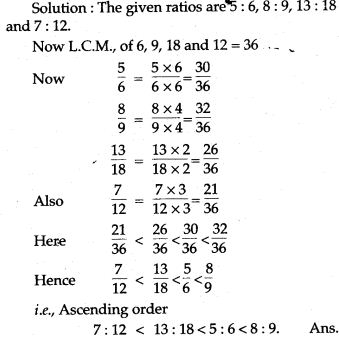Question 3. Find: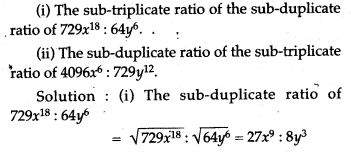Question 4. If 3x – 2y – 7z = 0 and 2x + 3y – 5z = 0 find x : y : z.Question 5. Two numbers are in the ratio of 3 : 5. If 8 is added to each number, the ratio becomes 2 : 3. Find the numbers.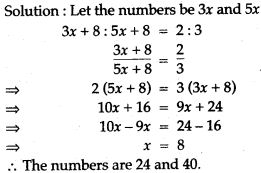Question 6. If x : y = 2 : 3, find the value of (3x + 2y) : (2x + 5y).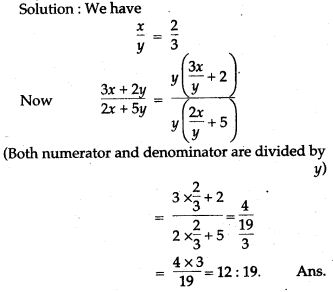Question 7. Divide Rs. 720 between Sunil, Sbhil and Akhil. So that Sunil gets 4/5 of Sohil’s and Akhil’s share together and Sohil gets 2/3 of Akhil’s share.Question 8. The ratio between two numbers is 3 : 4. If their L.C.M., is 180. Find the numbers.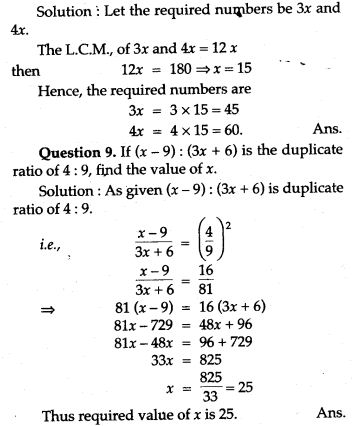Question 10. Find the compound ratio of the following:
(i) If A : B = 4 : 5, B : C = 6 : 7 and C : D = 14 : 15. Find A : D.
(ii) If P : Q = 6 : 7, Q : R = 8 : 9 find P : Q : R.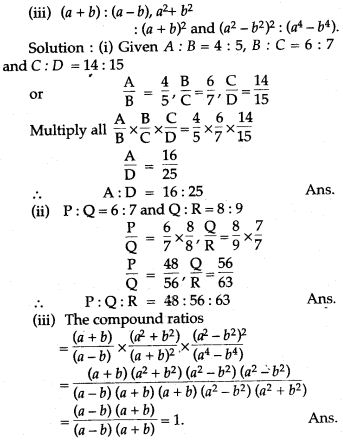Question 11. Find:
(i) The duplicate ratio of 7 : 9
(ii) The triplicate ratio of 3 : 7
(iii) The sub-duplicate ratio of 256 : 625
(iv) The sub-triplicate ratio of 216 : 343
(v) The reciprocal ratio of 8 : 15.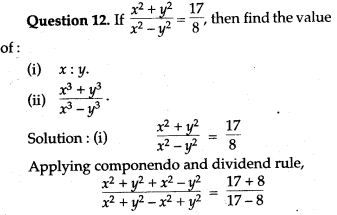Question 13. (i) What number must be added to each of the numbers 6, 15, 20 and 43 to make them proportional ?
(ii) What least number must be added to each of the numbers 5, 11, 19 and 37, so that they are in proportion ?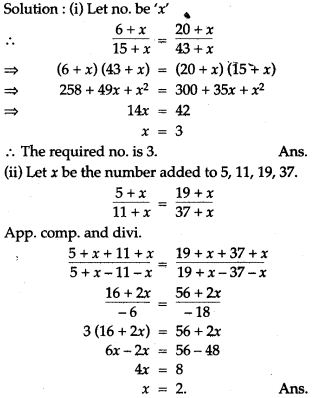Question 15. The work done by (x – 3) men in (2x + 1) days and the work done by (2x + 1) men in (x + 4) days are in the ratio of 3 : 10. Find the value of x.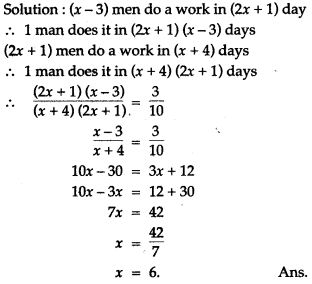Question 16. Find the third proportional to: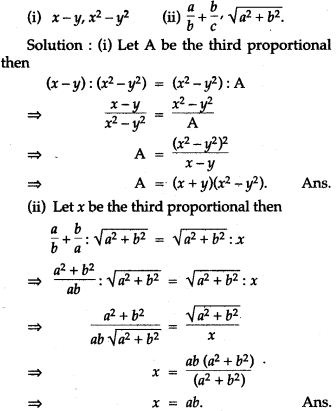Question 17. Find the fourth proportional to: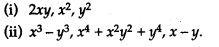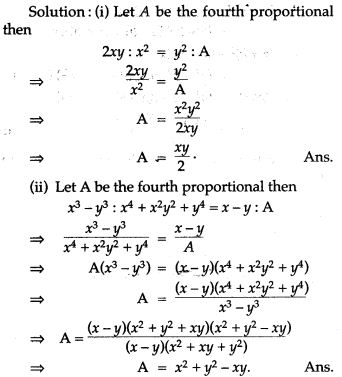Question 18. Find the two numbers such that their mean proprtional is 24 and the third proportinal is 1,536.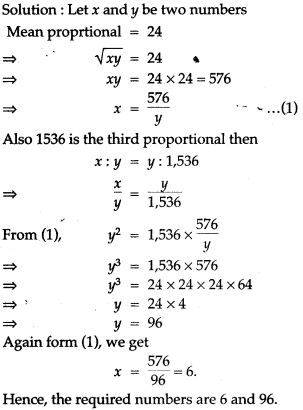Question 19. Using componendo and idendo, find the value of x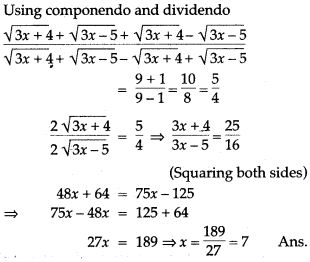Question 20. Solve for x: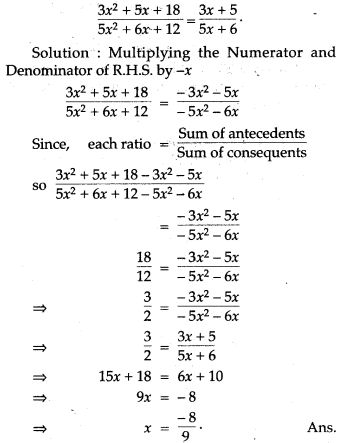Question 21. Using the properties of proportion, solve for x, given.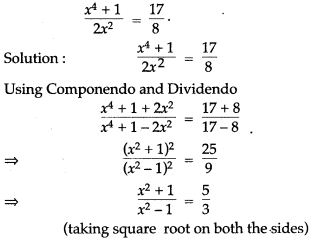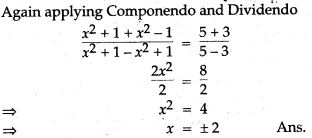Question 25. Find the value of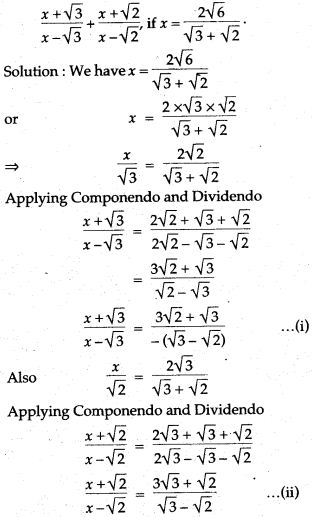### Prove the Following

Question 1. If (a – x) : (b – x) be the duplicate ratio of a : b show that: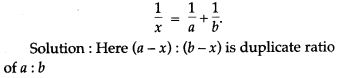Question 2. If a : b with a ≠ b is the duplicate ratio of a + c : b + c, show that c2 = ab.Question 3. If a : b = 5 : 3, show that (5a + 8b) : (6a – 7b) = 49 : 9.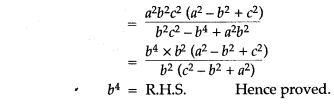Question 7. If x and y be unequal and x : y is the duplicate ratio of (x + z) and (y + z) prove that z is mean proportional between x and y.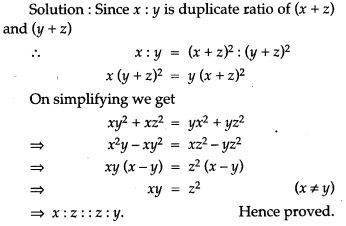Question 8. If ax = by = cz, prove that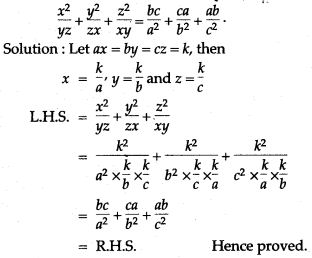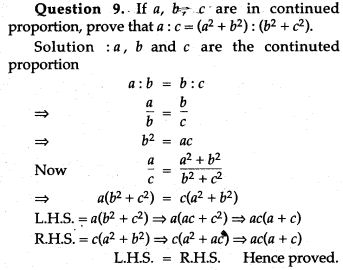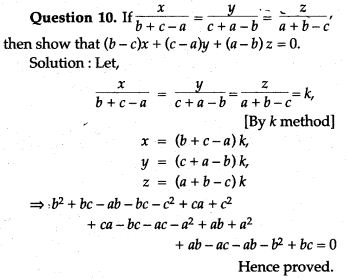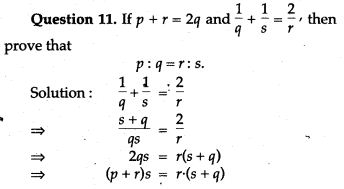Question 15. If a, b, c, d are in continued proportion, prove thatQuestion 16. If q is the mean proportional between p and r, prove that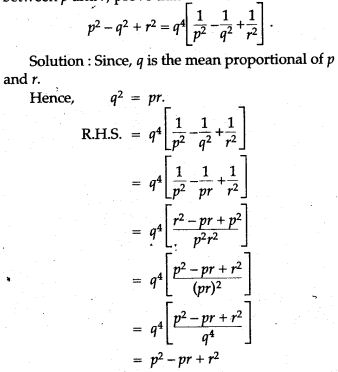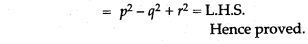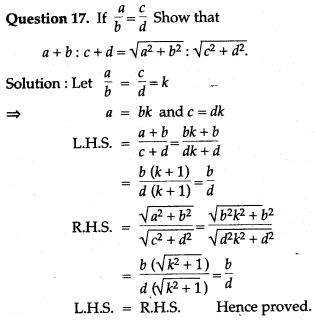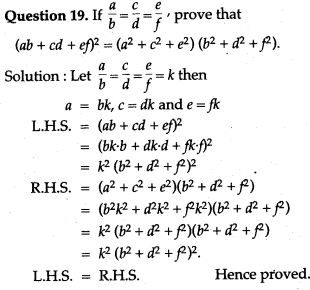Question 21. If a, b, c, d are in continued proportion, prove that: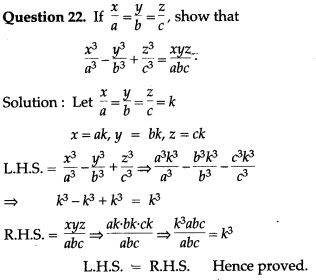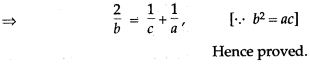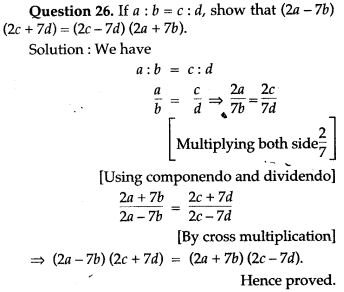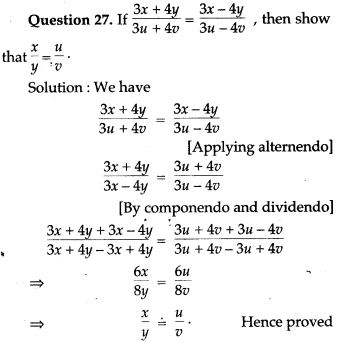For More Resources